# Lines toolbar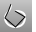Convert

Change a curve to polyline segments.CurveThroughPt

Draw a polyline (degree 1 curve) through points.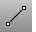Line

Draw a single line segment.LinesLine, Angled

Draw one angled line segment.Line, Angled, BothSides

Draw one angled line segment from its midpoint.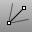Line, Bisector

Draw one line segment at a bisecting angle.Line, Bisector, BothSides

Draw one line segment at a bisecting angle from its midpoint.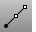Line, BothSides

Draw one line segment from its midpoint.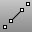Line, FourPoint

Draw one line segment by four points.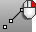Line, FourPoint, BothSides

Draw one line segment by four points from its midpoint.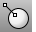Line, Normal

Draw a line segment normal to a surface.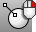Line, Normal, BothSides

Draw a line segment normal to a surface from its midpoint.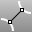Line, Perpendicular, 2Curves

Draw one line segment perpendicular to two curves.Line, Perpendicular From

Draw a line segment perpendicular from a curve.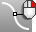Line, Perpendicular To

Draw a line segment perpendicular to a curve from its midpoint.Line, Tangent, 2Curves

Draw one line segment tangent to two curves.Line, Tangent From

Draw a line segment tangent from a curve.Line, Tangent, Perpendicular

Draw one line segment tangent and perpendicular to curves.LineThroughPt

Fit a line through any combination of points, control points, and point-cloud objects.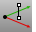Line, Vertical

Draw a line segment perpendicular to the construction plane.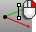Line, Vertical, BothSides

Draw a line segment perpendicular to the construction plane from its midpoint.Polyline

Draw a multi-segment polyline with options for line and arc segments, tracking line helpers, and close.PolylineOnMesh

Draw a multi-segment polyline on a mesh.

Rhinoceros 5 © 2010-2015 Robert McNeel & Associates. 17-Sep-2015